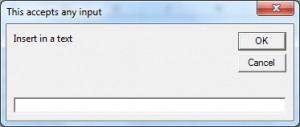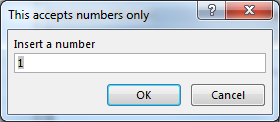# Decide what an INPUTBOX is supposed to return using VBA in Microsoft Excel

If you want to get any information from the user you can use the INPUTBOX-function.
This function displays a dialogbox that lets the user fill inn anything and returns the content as a string.
Sometimes you want to decide what the user is supposed to fill in, instead of accepting any information. That's when you use the INPUTBOX-method :

```Sub DecideUserInput()
Dim bText As String, bNumber As Integer
' here is the INPUTBOX-function :
bText = InputBox("Insert in a text", "This accepts any input")
' here is the INPUTBOX-method :
bNumber = Application.InputBox("Insert a number", "This accepts numbers only", 1)
MsgBox "You have inserted :" & Chr(13) & _
bText & Chr(13) & bNumber, , "Result from INPUT-boxes"
End Sub```

The INPUTBOX-method has a third argument, Type, that decides what the user is allowed to insert in the INPUTBOX-dialog.
Type can be one of the following :
Type :

```Accepted input:

0   A formula
1   A number
2   Text
4   A logical value (True or False)
8   A cell reference, e.g. a Range-object
16  An error value, e.g. #N/A
64  An array of values
```

When we run the macro we will get to see the following input boxes:In this way we can get the result through message box.

1.